1485字，阅读需时5分钟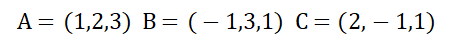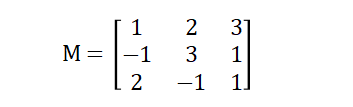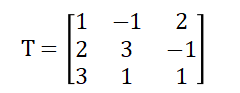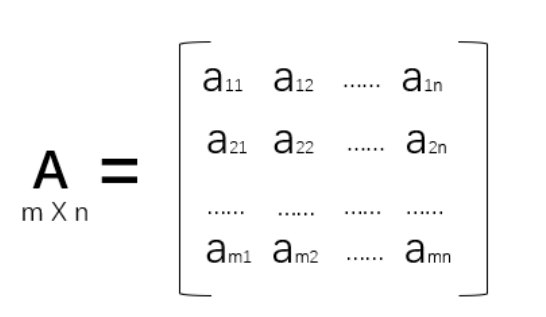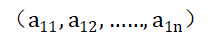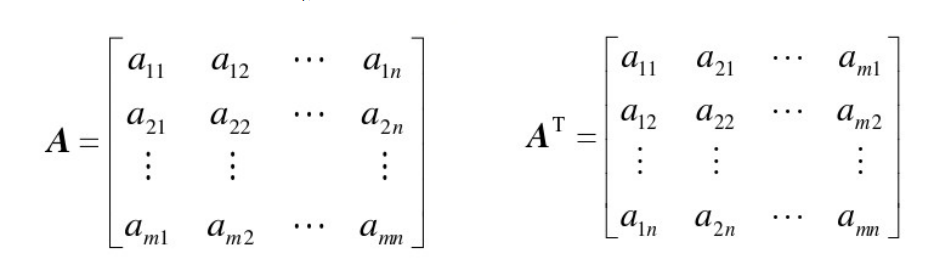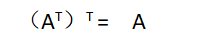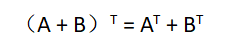A与B和的转置矩阵等于A的转置矩阵与B的转置矩阵的和。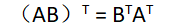A与B矩阵积的转置矩阵等于B的转置矩阵与A的转置的积（顺序不能颠倒）。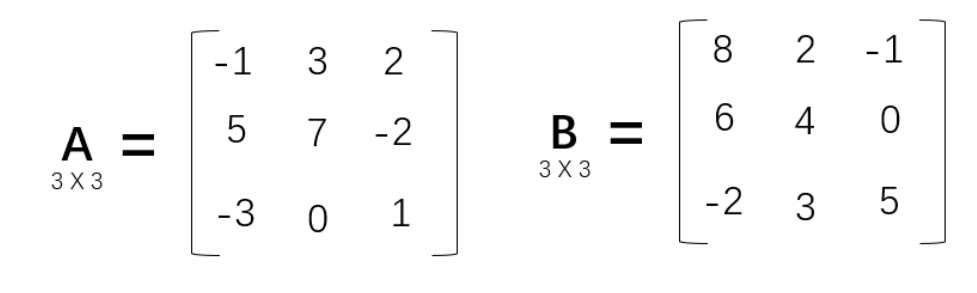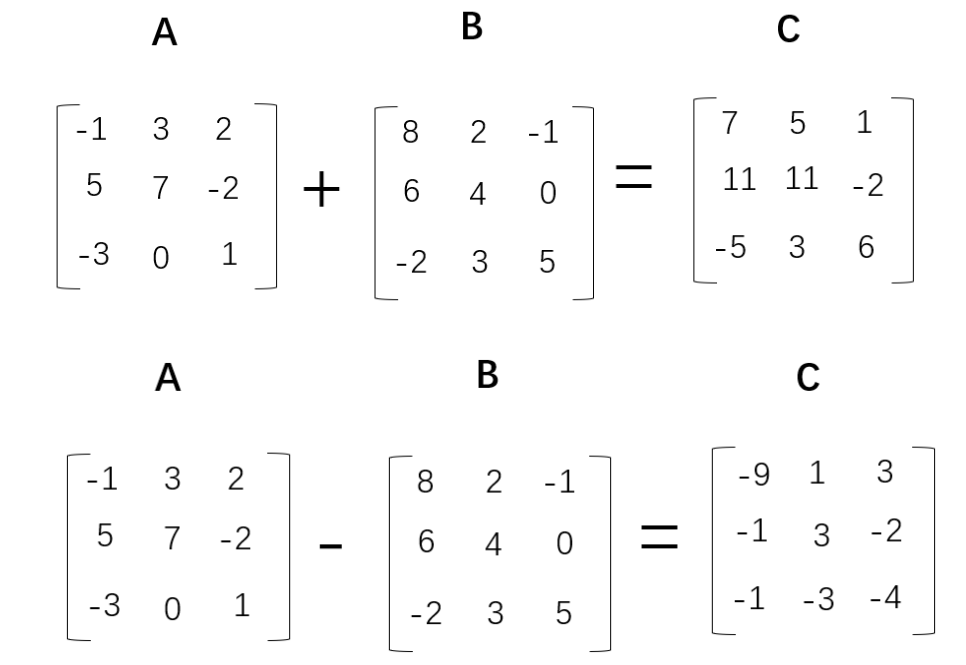（-1）* B的计算过程如下所示：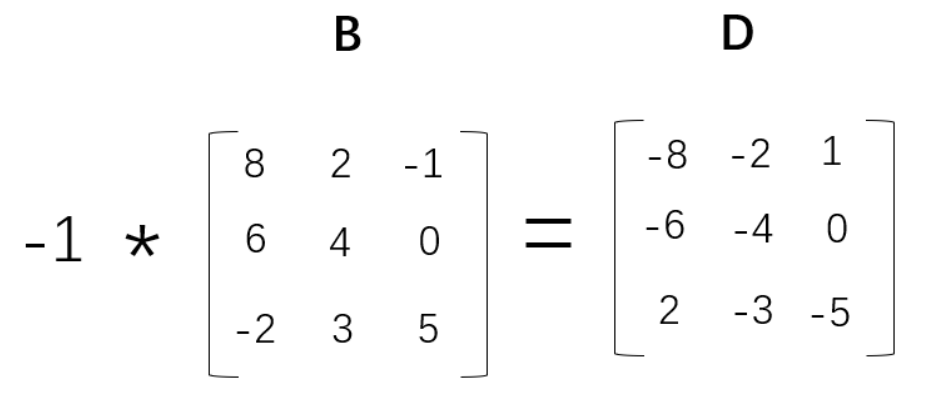#使用嵌套列表定义矩阵A和B
A = [[-1,3,2],[5,7,-2],[-3,0,1]]
B = [[8,2,-1],[6,4,0],[-2,3,5]]

#定义矩阵C，存储A+B的结果
C = [[0,0,0],[0,0,0],[0,0,0]]

#定义矩阵D，存储A-B的结果
D = [[0,0,0],[0,0,0],[0,0,0]]

# 遍历A矩阵的行
for i in range(len(A)):
# 遍历A矩阵的列
for j in range(len(A)):
C[i][j] = A[i][j] + B[i][j]
D[i][j] = A[i][j] - B[i][j]
print(C)
print(D)

# 导入numpy模块
import numpy as np

# 定义矩阵
A = np.array([[-1,3,2],[5,7,-2],[-3,0,1]])
B = np.array([[8,2,-1],[6,4,0],[-2,3,5]])

# 矩阵运算
print(A+B)
print(A-B)